Function Repository Resource:

# RationalInterpolation

Find a rational interpolation of a function

Contributed by: Wolfram Research
 ResourceFunction["RationalInterpolation"][expr,{x,m,n},{x1,x2,…,xn+m+1}] gives the rational interpolant to expr with numerator order m and denominator order n, where x1,x2,… are the abscissas of the interpolation points. ResourceFunction["RationalInterpolation"][expr,{x,m,n},{x,x0,x1}] gives the rational interpolant with the interpolation points chosen automatically on the interval x0 to x1.

## Details and Options

The following options can be given:
 WorkingPrecision MachinePrecision precision to use in internal computations "Bias" 0 bias in the automatic choice of interpolation points
Values for the "Bias" option must be numbers between -1 and 1. A positive value causes the points to be biased to the right and zero causes the points to be distributed symmetrically.

## Examples

### Basic Examples (2)

A rational interpolation of degree (2,4) to ex at seven equally spaced points between 0 and 2:

 In:=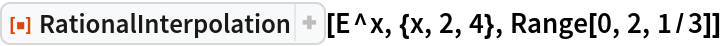Out=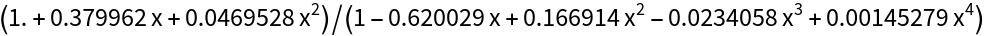The error between the function and the approximation tends to get larger near the endpoints:

 In:=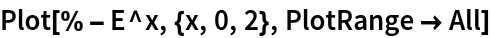Out=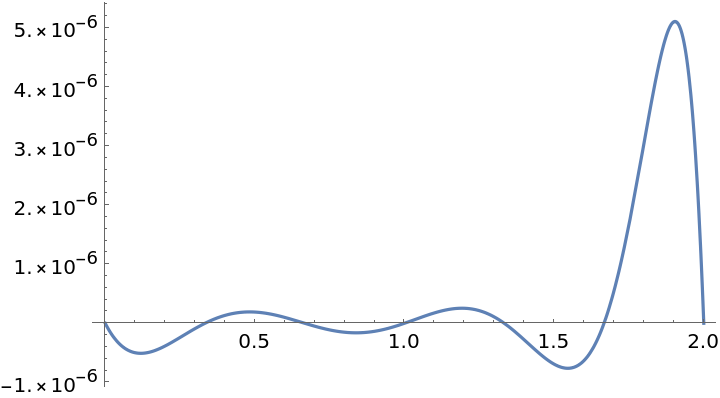Automatically choosing the interpolation points results in a smaller maximum error:

 In:=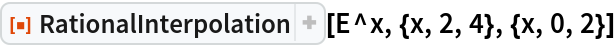Out=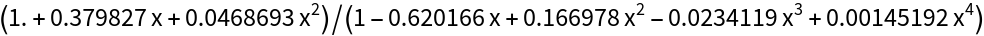In:=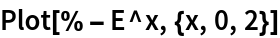Out=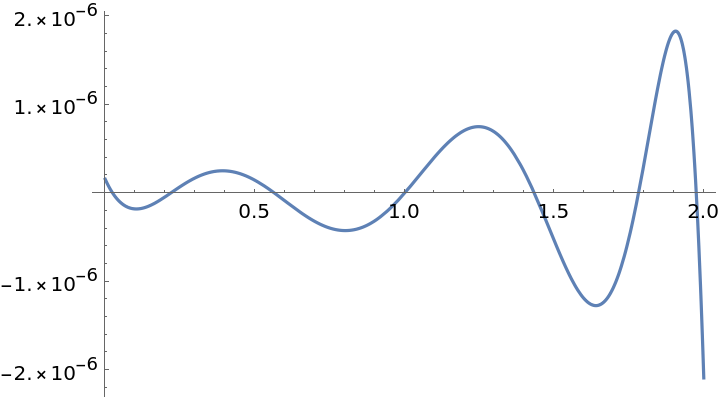### Options (1)

#### Bias (1)

Bias the distribution of the points to the right to get smaller errors there and larger errors to the left:

 In:=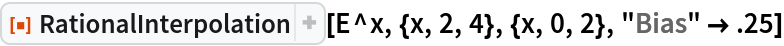Out=In:=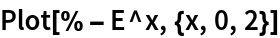Out=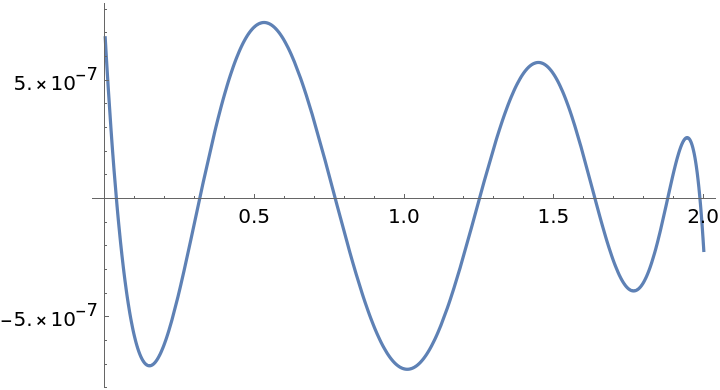## Requirements

Wolfram Language 11.3 (March 2018) or above

## Version History

• 1.0.0 – 29 March 2019